# 3D Vectors – vectors in 3-dimensional space

All of the things we have learned so far can be applied to 3D vectors. Essentially, we add the third dimension so that 3D vectors are now capable of describing points in space rather than just planes. Click here to revise 2D vectors including unit vectors and how to find magnitude and direction. Also recall 2D vector arithmetic and vectors in context such as position vectors and vectors in trigonometry. At this level, we often extend a lot of the earlier mechanics problems to two dimensions – it is very simple to add the third dimension. See Calculus in Kinematics, for example.

## Unit 3D Vectors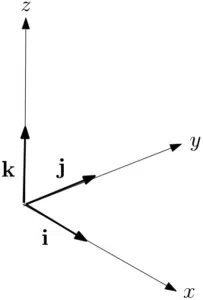Recall the unit vectors $\hspace{5pt}{\bf i}=\left(\begin{array}{c}1\\0\end{array}\right)$ and $\hspace{5pt}{\bf j}=\left(\begin{array}{c}0\\1\end{array}\right)$ when working in two dimensions. We now extend to three dimensions and we introduce the third unit vector:

${\bf i}=\left(\begin{array}{c}1\\0\\0\end{array}\right),\hspace{20pt} {\bf j}=\left(\begin{array}{c}0\\1\\0\end{array}\right),\hspace{20pt}{\bf k}=\left(\begin{array}{c}0\\0\\1\end{array}\right)$

These vectors point in the $x$, $y$ and $z$ directions respectively. Notice the position of the $x$ and $y$ axes relative to the $z$-axis. We call this the right-hand rule. This means that if the $x$-axis is your index finger on your right hand and the $y$-axis is your middle finger, then the $z$-axis is your thumb and its points upwards. We often see it in different orientations (see Example 1) but usually with the $z$-axis pointing upwards. If you find that with your thumb pointing upwards, the $x$ and $y$ axis are on the wrong fingers, you are likely using a left-handed coordinate frame.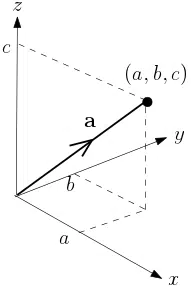With these vectors, we can now identify the position vector that points from the origin to any point in 3D space. The vector ${\bf a}$ we can see here is a position vector as it points from the origin. We can write

${\bf a}=\left(\begin{array}{c}a\\b\\c\end{array}\right)=a{\bf i}+b{\bf j}+c{\bf k}$.

It follows, by a simple application of 3D Pythagoras, that the magnitude of this vector is

$\vert {\bf a}\vert= \sqrt{a^2+b^2+c^2}$.

It follows that a unit vector in the direction of ${\bf a}$ is  given by $\hat{\bf a}=\frac{{\bf a}}{\vert{\bf a}\vert}$. See Example 1.

## 3D Vector Arithmetic and Magnitude

We can apply the operations that we saw on the vector arithmetic page in a similar fashion. That is, suppose we have the vectors ${\bf a}=\left(\begin{array}{c}a_1\\a_2\\a_3\end{array}\right)=a_1{\bf i}+a_2{\bf j}+a_3{\bf k}$ and ${\bf b}=\left(\begin{array}{c}b_1\\b_2\\b_3\end{array}\right)=b_1{\bf i}+b_2{\bf j}+b_3{\bf k}$. It follows that

${\bf a}+{\bf b}=\left(\begin{array}{c}a_1\\a_2\\a_3\end{array}\right)+\left(\begin{array}{c}b_1\\b_2\\b_3\end{array}\right)=\left(\begin{array}{c}a_1+b_1\\a_2+b_2\\a_3+b_3\end{array}\right)=(a_1+b_1){\bf i}+(a_2+b_2){\bf j}+(a_3+b_3){\bf k}$

${\bf a}-{\bf b}=\left(\begin{array}{c}a_1\\a_2\\a_3\end{array}\right)-\left(\begin{array}{c}b_1\\b_2\\b_3\end{array}\right)=\left(\begin{array}{c}a_1-b_1\\a_2-b_2\\a_3-b_3\end{array}\right)=(a_1-b_1){\bf i}+(a_2-b_2){\bf j}+(a_3-b_3){\bf k}$

$m{\bf a}=m\left(\begin{array}{c}a_1\\a_2\\a_3\end{array}\right)=\left(\begin{array}{c}ma_1\\ma_2\\ma_3\end{array}\right)=ma_1{\bf i}+ma_2{\bf j}+ma_3{\bf k}\hspace{20pt}$ where $m$ is a scalar constant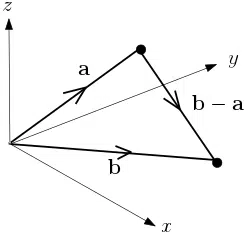We can easily extend the geometric interpretations that we saw on the vector arithmetic page to 3 dimensions. For example, the vector between two points in 3D is given by ${\bf b}-{\bf a}=(b_1-a_1){\bf i}+(b_2-a_2){\bf j}+(b_3-a_3){\bf k}$. It follows that the distance between these two points is the magnitude of this vector and given by $\vert {\bf b}-{\bf a}\vert =\sqrt{(b_1-a_1)^2+(b_2-a_2)^2+(b_3-a_3)^2}$. Note that ${\bf b}-{\bf a}$ is not a position vector as it doesn’t point from the origin. It does however lie in the same plane as ${\bf a}$ and ${\bf b}$. We say that ${\bf a}$, ${\bf b}$ and ${\bf b}-{\bf a}$ are coplanar. See Example 1.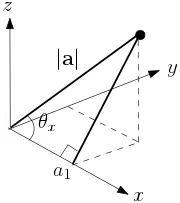We can also extend the concept of direction to 3D vectors. A 3D vector now lies in space and not on a plane but a simple application of SOHCAHTOA (cos=adj/hyp) tells us that $\cos(\theta_x)=\frac{a_1}{\vert {\bf a}\vert}$ for the vector ${\bf a}=a_1{\bf i}+a_2{\bf j}+a_3{\bf k}$ that makes an angle of $\theta_x$ with the $x$-axis. Similarly, $\cos(\theta_y)=\frac{a_2}{\vert {\bf a}\vert}$ and $\cos(\theta_z)=\frac{a_3}{\vert {\bf a}\vert}$ where ${\bf a}$ makes an angle of $\theta_y$ and $\theta_z$ with the $y$ and $z$ axes respectively. See Example 2.

## Example of 3D Vectors

The points $A$ and $B$ have position vectors ${\bf a}=-{\bf i}-2{\bf j}-{\bf k}$ and ${\bf b}=2{\bf i}-4{\bf j}+3{\bf k}$ respectively.

1. Find the exact distance between the points $A$ and $B$.
2. Show that the triangle $\triangle OAB$ is isosceles.
3. The point $C$ is such that the position vector $\overrightarrow{OC}=2{\bf a}+5{\bf b}$. Find a unit vector in the direction $\overrightarrow{BC}$.

The diagram shows a cube with sides of length 1 and vertices at $O$, $A$, $B$, $C$, $D$, $E$, $F$ and $G$.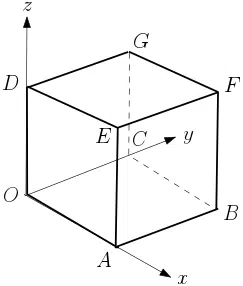1. Express the diagonal $\overrightarrow{OF}$ in vector notation.
2. Find the cosine of the angle that the diagonal makes with the $y$-axis.